# Counting motifs in the human interactome

## Abstract

Small over-represented motifs in biological networks often form essential functional units of biological processes. A natural question is to gauge whether a motif occurs abundantly or rarely in a biological network. Here we develop an accurate method to estimate the occurrences of a motif in the entire network from noisy and incomplete data, and apply it to eukaryotic interactomes and cell-specific transcription factor regulatory networks. The number of triangles in the human interactome is about 194 times that in the Saccharomyces cerevisiae interactome. A strong positive linear correlation exists between the numbers of occurrences of triad and quadriad motifs in human cell-specific transcription factor regulatory networks. Our findings show that the proposed method is general and powerful for counting motifs and can be applied to any network regardless of its topological structure.

## Introduction

The increasing availability of genomic and proteomic data has propelled network biology to the frontier of biomedical research1,2,3,4. Network biology uses a graph to depict interactions between cellular components (proteins, genes, metabolites and so on), where the nodes are cellular components and the links represent interactions. Two of the most surprising discoveries from the genome sequencing projects are that the human gene repertoire is much smaller than had been expected, and that there are just over 200 genes unique to human beings5. As the number of genes alone does not fully characterize the biological complexity of living organisms, the scale of physiologically relevant protein and gene interactions are now being investigated to understand the basic biological principles of life6,7,8. Although the list of known protein–protein interactions (PPIs) and gene regulatory interactions (GRIs) is expanding at an ever-increasing pace, the human PPI and GRI networks are far from being complete and, hence, their dynamics have yet to be uncovered9,10,11.

The feed-forward loop (FFL) and several other graphlets (called motifs) are found to be over-represented in different biological networks11. Furthermore, over-represented motifs usually represent functional units of biological processes in cells. Hence, it is natural to ask whether a motif, such as a triangle, appears more often in the interactome of humans than in that of other species, or whether the FFL or the bi-fan appears more frequently in the human gene regulatory network. As the biological networks that have been reported are actually the subnetworks of the true ones and often contain remarkably many incorrect interactions for eukaryotic species, there are two approaches to answering these questions. One approach is to infer spurious and missing links in the entire network12,13,14, and then to count motif occurrences. Another approach is to estimate the number of motif occurrences in the interactome from the observed subnetwork data using the same method as that for estimating the size of eukaryotic interactomes9,10,15. If we have the number of occurrences of a motif or its estimate in a network, we can determine whether the motif is over-represented or not, based on how often the motif is seen in a random network with similar structural parameters11,16,17.

In the present work, we take spurious and missing link errors into account to develop an unbiased and consistent estimator for the motif count. The method works for both undirected and directed networks. We derive explicit mathematical expressions for the estimators of five commonly studied triad and quadriad network motifs (Fig. 1). These estimators are further validated extensively for each of the following four models: Erdös–Renyi (ER)18, preferential attachment19, duplication20 and the geometric model21 (Supplementary Note 1). By applying the method to eukaryotic interactomes, we find that the number of triangles in the human interactome is about 194 times that of the Saccharomyces cerevisiae interactome, three times as large as expected. By applying the method to human cell-specific transcription factor (TF) regulatory networks22, we discover a strong positive linear correlation between the counts of widely studied triads and quadriads. We also notice that the embryonic stem cell’s TF regulatory network has the smallest number of occurrences relative to its network size for all the five motifs under study.

## Results

### Estimating motif occurrences

In this study, we shall consider PPIs and gene regulatory networks. The former are undirected, whereas the latter are directed networks. Consider a directed or undirected network (V, E), where V is the set of nodes and E is the set of links. For simplicity, we assume that has n nodes and V={1,2,3,…,n}. Let obs(Vobs, Eobs) be an observed subnetwork of . Following (ref. 9), we model an observed subnetwork as the outcome of a uniform node sampling process in the following sense. Let Xi be independent and identically distributed Bernoulli random variables with the parameter p(0,1] for i=1,2,…,n. We use Xi=1 and Xi=0 to denote the events that node i is sampled and not sampled, respectively. Then Vobs is the set of nodes i with Xi=1, and Eobs is induced from E by Vobs. For clarity of presentation, we first introduce our method for the case when the observed subnetwork is free from experimental errors, and then generalize it to handle noisy observed subnetwork data.

Consider a motif . We use N and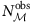to denote the number of occurrences of in and obs, respectively. We assume that the number of nodes, n, is known, but only links in Gobs are known. We are interested in estimating N from the observed subnetwork obs. As obs is assumed to be free from experimental errors, we can obtainsimply by enumeration. Let us define the following: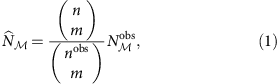where m and nobs are the number of nodes in and obs, respectively.

Let A=[aij]1≤i,jn denote the adjacency matrix of , where aij=1 if there is a link from i to j, and aij=0 otherwise. Furthermore, for a subset J{1,2,…,n}, A[J] denotes the submatrix consisting of entries in the rows and columns indexed by J. We write N as a function of A andas a function of A and the random variables Xi. We also assume the following: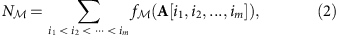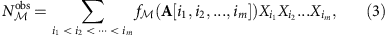where f() is a function chosen to decide whether occurs among nodes i1,i2,…,im or not. For the motifs listed in Table 1, their corresponding functions f() are given in Supplementary Table S1.

From equations (1) and (3), we have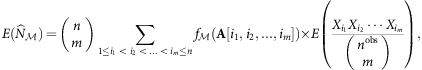where nobs is a random variable such that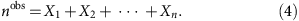As the random variables Xi are independent and identically distributed, for any 1≤i1<i2<…<imn, we also have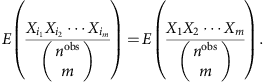Hence, by equation (2),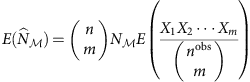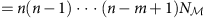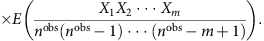By conditioning on the event that X1=X2=···=Xm=1, we rewrite equation (4) aswhere ZBinomial(nm,p), and hence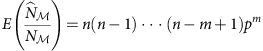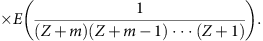As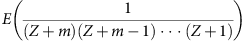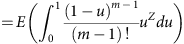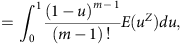we have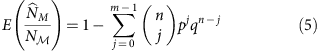by applying integration by parts and simplification. Therefore, we have obtained the following theorem.

Theorem 1: Let be a network of n nodes. Assume obs is a subnetwork of obtained by a uniform node sampling process that selects a node with probability p. For any motif of m nodes, the estimator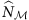defined in equation (1) satisfies equation (5). Therefore,is an asymptotically unbiased estimator for N in the sense that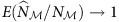as n goes to infinity. Moreover, the convergence is exponentially fast in n.

When the estimator (1) is applied to estimate the number of links in an undirected network , the variance has the following closed-form expression: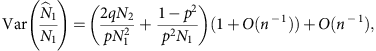where N1 and N2 are, respectively, the number of links and three-node paths in (Supplementary Methods). This leads to our next theorem.

Theorem 2: When is generated from one of the ER, preferential attachment, duplication or geometric models,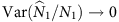as n goes to infinity.

Theorem 2 says that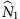is consistent. For an arbitrary motif, it is much more complicated to derive the variance of the estimator (1). Nevertheless, our simulation shows that for all the motifs in Fig. 1, the variance of the estimator converges to zero as n goes to infinity and, hence, it is consistent (Fig. 2 and Supplementary Figs S1–S8). We wish to point out that the notions ‘asymptotically unbiased’ and ‘consistent’ are not used in the usual statistical sense where the population is fixed and the number of observations increases to infinity.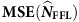Figure 2: Plots of and for counting the occurrences of FFL.

For realistic estimation, one has to take error rates into account, as detecting PPIs or GRIs is error prone to some degree. PPIs or gene regulatory networks have spurious interactions (that is, false positives) and missing interactions (that is, false negatives). We define the false-positive rate r+ to be the probability that a non-existing link is incorrectly reported, and the false-negative rate r to be the probability that a link is not observed. Using the independent random variables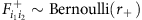and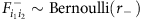to model spurious and missing interactions in the observed subnetwork obs, we can represent the effect of experimental errors on an ordered pair of nodes (i1,i2) asIn other words, for any two nodes i1,i2Vobs, a link (i1,i2) is observed in the subnetwork obs (that is,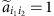) if (i) there is a link (i1,i2) in the real network (that is, $a i 1 i 2$=1) and there is no false negative (that is,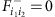) or (ii) the link (i1,i2) does not exist in the real network (that is, $a i 1 i 2$=0) but a false positive occurs (that is,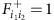).

To take error rates into account, we simply replace each entry ai1,i2 in the adjacency matrix A with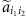to obtain a new matrix,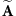, and then replace A within equation (3). For any motif in Table 1, the expectation of the estimatorin equation (1) can be expressed as (Supplementary Methods)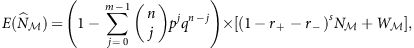where s is the number of links that has and W is a function of n, r, r+, and N for all proper submotifs ′ of (Supplementary Table S2). Thus, to correct the bias caused by link errors, we derive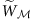from W by replacing N with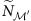for all submotifs of , and use the following formula to estimate N: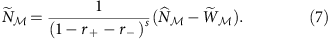For the motifs listed in Fig. 1, the corresponding bias-corrected estimators are given in Table 1.

We examined the accuracy of the proposed estimators on networks generated by a random network model. As these estimators are asymptotically unbiased, we used the mean square error (MSE) of the ratios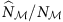and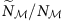, defined later in equation (9), to measure their accuracy (see Methods section). Figure 2 summarizes the simulation results for the FFL motif in random networks generated from the preferential attachment model19. (The results for other motif network model combinations are similar and can be found in Supplementary Figs S1–S8.) First, when the edge density ρ is fixed, the MSE of the estimators for FFL decreases and converges to zero as n increases (Fig. 2a,b). Second, the MSE decreases as the edge density increases, suggesting that the estimators are even more accurate when applied to less sparse networks. Third, the MSE of the estimators decreases as p increases (Fig. 2c). Finally, the MSE increases with r and r+ (Fig. 2d). Altogether, our simulation tests confirm that the proposed estimators are accurate for any underlying network.

### Motif richness in the human interactome

The entire interactomes for eukaryotic model organisms such as S. cerevisiae, Caenorhabditis elegans, Homo sapiens and Arabidopsis thaliana are not fully known. We estimated the interactome size (that is, the number of interactions) and the number of triangles in the entire PPI network for S. cerevisiae, C. elegans, H. sapiens and A. thaliana, using the data sets CCSB-YI1 (ref. 23), CCSB-WI-2007 (ref. 24), CCSB-HI1 (refs 25, 26) and CCSB-AI1-Main27. These data sets were produced from yeast two-hybrid experiments and their quality parameters are summarized in Table 2 for convenience.

First, we re-estimated the size of four interactomes using the bias-corrected estimator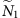(Table 1). To test all possible interactions between selected proteins, the sets of bait and prey proteins should be exchanged in the two rounds of interaction mating in a high-throughput yeast two-hybrid experiment28. However, this is only true for the C. elegans and H. sapiens data sets (CCSB-WI-2007 and CCSB-HI1, respectively). For the S. cerevisiae and A. thaliana data sets (CCSB-YI1 and CCSB-AI1-Main, respectively), the set of bait proteins are slightly different from the set of prey proteins. For these two cases, we applied our estimator to the subnetwork induced by the intersection of the bait and prey protein sets.

The following estimator was proposed by Stumpf et al.9 for the size of an interactome and was later used to estimate the size of the eukaryotic interactomes23,24,26,27: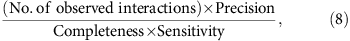where ‘completeness’ is the fraction of all possible pairwise protein combinations that have been tested. In our notation,

(No. of observed interactions)=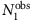,

Sensitivity=1−r,

Precision=1−rd,

Completeness=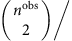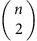, where rd is the proportion of spurious links among detected links and is called the false discovery rate. (Note that rd was called the false-positive rate in ref. 9.) Thus, the estimator (8) becomes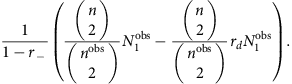For PPI data sets, r+ is about 10−4 and thus 1−r≈1−rr+. As rd is also small, our estimatorhandles errors differently but is quite close to the estimator (8). In particular, when the precision is 100% or, equivalently, when rd=r+=0, these two estimators are equal (Supplementary Note 2 and Supplementary Fig. S9). Indeed, our estimates for interactome size agree well with those obtained from equation (8) (Table 2). Such an agreement demonstrates again that our estimators for counting motifs are accurate.

We proceed further to estimate the number of triangles in each of the interactomes using the corresponding bias-corrected estimator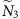in Table 1. For each interactome, we estimated the number of triangles from the observed subnetwork data directly and from sampling the observed subnetwork repeatedly. The two estimates agree well (Table 2).

Our estimation shows that although the size of the A. thaliana interactome is about 1.8 times that of the human interactome, it contains fewer triangles than the human interactome does. The triangle density of the human and C. elegans interactomes are similar and are 1.7 times that of the A. thaliana and 5 times that of S. cerevisiae. The size of the human interactome is only 15 times that of the S. cerevisiae interactome, yet the number of triangles in the former is about 194 times that in the latter, 3 times as large as expected.

### Correlation between motif counts in TF regulatory networks

Recently, the TF regulatory networks of 41 human cell and tissue types were obtained from genome-wide in vivo DNasel footprints map22. In these networks, the nodes are 475 TFs and the regulation of each TF by another is represented by network-directed links. Motif count analysis showed that the distribution of the motif count is unimodal, with the peak corresponding to the mean value for each motif (diagonal panels in Fig. 3). Surprisingly, there is a very strong linear correlation between the counts in the TF regulatory networks of different cell types, even for the triad and quadriad motifs that are topologically very different (Fig. 3).Figure 3: Correlation of motif counts in 41 human cell-specific TF regulatory networks.

Given that human has about 2,886 TF proteins29, we further estimated the number of occurrences of the 5 motifs for each of the 7 functionally related classes of cells (Fig. 3 and Table 3). This was achieved by simply setting the false-positive and -negative rates to 0, as they are currently unknown. The TF regulatory networks of blood cells have diverse motif counts. Specifically, for all triad and quadriad motifs, the promyelocytic leukemia cell TF regulatory network has the largest number of occurrences, whereas the erythoid cell TF regulatory network has the smallest number of occurrences. The embryonic stem cell TF regulatory network has the smallest number of occurrences relative to its network size for all the motifs.

In a random network, the ratio of the FFL count to the feedback loop count is 3:1. However, in the human cell-specific TF regulatory networks, the ratio is about 10:1, suggesting FFL is significantly enriched in these networks. Table 3 also suggests that the bi-fan motif is relatively abundant in these networks, as the ratio of the bi-fan count to the biparallel count is roughly 1:2 in a random network.

## Discussion

By taking spurious and missing link rates into account, we have developed a powerful method for estimating the number of motif occurrences in the entire network from noisy and incomplete data for the first time. It extends previous studies on interactome size estimation9,10,23,24,25,26,27 to motif count estimation in a directed or undirected network. Such a method is important because exact motif enumeration is possible only if the network is completely known, which is often not the case in biology. Our proposed method has been proven mathematically as being unbiased and accurate without any assumption at all regarding the topological structure of the underlying networks. Therefore, our proposed estimators can be applied to all the widely studied networks in social, biological and physical sciences.

Interactome size has been estimated from noisy subnetwork data by using equation (8), where the precision (which is 1−rd) and sensitivity of the data are taken into account23,24,26,27. This approach might yield an inaccurate estimate, as the false discovery rate is often calculated from gold-standard data sets30,31,32,33 and can be quite unreliable, as indicated in ref. 26, in which the false discovery rate for the data set CCSB-HI1 was adjusted from 87% to 93%, to 20.6%, after multiple cross-assay validation. By contrast, our proposed method uses false-positive and false-negative rates for motif count estimation. As the false-negative rate is equal to 1−sensitivity and the false-positive rate is only about 10−4, our method is more robust than estimations based on the false discovery rate.

Theorems 1 and 2 in the present paper show that motif counting via sampling and then scaling up in a huge network is not merely fast but can also give accurate estimate. Take the triangle motif, for instance. In our validation test, the equation (1)-based sampling achieved less than 1% deviation from the actual count by using no more than 50% of the computing time compared with the naive triangle counting method (Fig. 4 and Supplementary Note 3). As the obtained sampling approach takes positive and negative link-error rates into account, it is a good addition to the methodology for estimating motif count in networks34,35.

By applying our estimation method to PPI subnetwork data for four eukaryotic organisms, we found that the numbers of triangles in a eukaryotic interactome differ considerably. For example, the triangle motif is exceptionally enriched in the human interactome. As noted in ref. 9, we have to keep in mind that the estimates in Table 2 are based on PPIs that are detectable, given current experimental methods. However, our estimators will remain correct for any interaction data available in the future.

We also discovered that there is a very strong positive linear correlation between triad and quadriad motif occurrences in human cell-specific TF regulatory networks, and that the TF regulatory network of embryonic stem cells has the smallest number of occurrences relative to its network size for each of the common triad and quadriad motifs. Hence, our study reveals a surprising feature of the TF regulatory network of embryonic stem cells.

Finally, we remark that the proposed estimators for motif counting are derived using the assumption that the subnetwork data is the outcome of a uniform node sampling process. In practice, however, biologists may select proteins for study according to their biological importance. The accuracy of our proposed method was examined for a degree-bias and two other non-uniform node sampling schemes (Supplementary Note 4 and Supplementary Figs S10–S12). In the degree-bias sampling process, a network node is sampled independently with a probability that is proportional to its degree in the underlying network. By the nature of this sampling process, it leads to overestimation of motif count when our proposed estimator is used. Our simulation tests indicate that its effect on the estimation of motif count depends on the scale-free structure of the underlying network and the proportion of the sampled nodes. In particular, when more than 60% of nodes in a network are sampled, the estimate is no more than five times the actual count. Hence, the triangle counts in the four eukaryotic interactomes are likely less than the estimates listed in Table 2 by a small constant factor. How to correct the overestimation caused by a degree-bias node sampling is challenging and worthy to study in future.

## Methods

### Interaction data

Human, yeast, worm and A. thaliana PPI data sets were downloaded from the Center for Cancer Systems Biology (CCSB) ( http://ccsb.dfci.harvard.edu): CCSB-YI1 (ref. 23), CCSB-WI-2007 (ref. 24), CCSB-HI1 (refs 25, 26 and CCSB-AI1-Main27. TF regulatory interaction data sets were downloaded from the Supplementary Information of ref. 22 in the Cell journal website.

### Simulation validation for motif estimators

We considered four widely used random graph models: ER18, preferential attachment19, duplication20 and geometric models21 (Supplementary Note 1). Using each model, we generated 200 random networks by using different combinations of node number n{500,1,000,1,500,…,10,000} and edge density ρ{0.01,0.02,…,0.1}. Each generated network was taken as the whole network , from which 100 subnetworks were sampled using the node sampling probability p{0.05,0.1,0.15,…,0.5}. For each motif appearing in Fig. 1, we first computed(given in equation (1)) from the motif count in each sampled subnetwork. This was used as an estimate of the number of occurrences of the motif in the error-free case, N. Spurious and missing interactions were then planted in the sampled subnetworks with the chosen error rates r+ and r. The bias-corrected estimator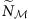(given in Table 1) for N was then recalculated. We used the MSE of the ratiosandto measure the consistency (and hence accuracy) ofand, respectively.

For the estimator Y of a parameter θ, the MSE of Y in estimating θ is defined as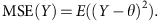This expression can be used to measure the MSE made in the estimation. In our validation test, we sampled 100 subnetworks from a network to evaluate the consistency of the estimatorof a motif . As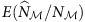approaches to 1 when n is large (Theorem 1), the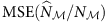was approximately computed as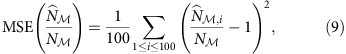where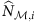is the estimate calculated from the ith subnetwork using, 1≤i≤100. Computing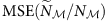is similar.

How to cite this article: Tran, N. H. et al. Counting motifs in the human interactome. Nat. Commun. 4:2241 doi: 10.1038/ncomms3241 (2013).

## References

1. 1

Barabasi, A.-L. & Oltvai, Z. N. Network biology: understanding the cell’s functional organization. Nat. Rev. Genet. 5, 101–113 (2004).

2. 2

Ideker, T., Dutkowski, J. & Hood, L. Boosting signal-to-noise in complex biology: prior knowledge is power. Cell 144, 860–863 (2011).

3. 3

Vidal, M., Cusick, M. E. & Barabasi, A.-L. Interactome networks and human disease. Nat. Rev. Genet. 12, 56–68 (2011).

4. 4

Barabasi, A.-L., Gulbahce, N. & Loscalzo, J. Network medicine: a network-based approach to human disease. Cell 144, 986–998 (2011).

5. 5

International Human Genome Sequencing Consortium. Finishing the euchromatic sequence of the human genome. Nature 431, 931–945 (2004).

6. 6

Jeong, H., Mason, S. P., Barabasi, A.-L. & Oltvai, Z. N. Lethality and centrality in protein networks. Nature 411, 41–42 (2001).

7. 7

Hahn, M. W. & Kern, A. D. Comparative genomics of centrality and essentiality in three eukaryotic protein- interaction networks. Mol. Biol. Evol. 22, 803–806 (2004).

8. 8

He, X. & Zhang, J. Why do hubs tend to be essential in protein networks? PLoS Genet. 2, e88 (2006).

9. 9

Stumpf, M. P. H. et al. Estimating the size of the human interactome. Proc. Natl Acad. Sci. USA 105, 6959–6964 (2008).

10. 10

Rottger, R., Ruckert, U., Taubert, J. & Baumbach, J. How little do we actually know? On the size of gene regulatory networks. IEEE/ACM Trans. Comput. Biol. Bioinform. 9, 1293–1300 (2012).

11. 11

Milo, R. et al. Network motifs: simple building blocks of complex networks. Science 298, 824–827 (2002).

12. 12

Deng, M., Mehta, S., Sun, F. & Chen, T. Inferring domain-domain interactions from protein-protein interactions. Genome Res. 12, 1540–1548 (2002).

13. 13

Liu, Y., Liu, N. & Zhao, H. Inferring protein-protein interactions through high-throughput interaction data from diverse organisms. Bioinformatics 21, 3279–3285 (2005).

14. 14

Guimera, R. & Sales-Pardo, M. Missing and spurious interactions and the reconstruction of complex networks. Proc. Natl Acad. Sci. USA 106, 22073–22078 (2009).

15. 15

Sambourg, L. & Thierry-Mieg, N. New insights into protein-protein interaction data lead to increased estimates of the S. cerevisiae interactome size. BMC Bioinformatics 11, 605 (2010).

16. 16

Kashtan, N., Itzkovitz, S., Milo, R. & Alon, U. Efficient sampling algorithm for estimating subgraph concentrations and detecting network motifs. Bioinformatics 20, 1746–1758 (2004).

17. 17

Picard, F., Daudin, J.-J., Koskas, M., Schbath, S. & Robin, S. Assessing the exceptionality of network motifs. J. Comput. Biol. 15, 1–20 (2008).

18. 18

Erdos, P. & Renyi, A. On the strength of connectedness of a random graph. Acta Math Hung. 12, 261–267 (1960).

19. 19

Barabasi, A.-L. & Albert, R. Emergence of scaling in random networks. Science 286, 509–512 (1999).

20. 20

Chung, F., Lu, L., Dewey, T. G. & Galas, D. J. Duplication models for biological networks. J. Comput. Biol. 10, 677–687 (2003).

21. 21

Przulj, N., Corneil, D. G. & Jurisica, I. Modeling interactome: scale-free or geometric? Bioinformatics 20, 3508–3515 (2004).

22. 22

Neph, S. et al. Circuitry and dynamics of human transcription factor regulatory networks. Cell 150, 1274–1286 (2012).

23. 23

Yu, H. et al. High-quality binary protein interaction map of the yeast interactome network. Science 322, 104–110 (2008).

24. 24

Simonis, N. et al. Empirically controlled mapping of the Caenorhabditis elegans protein–“protein interactome network. Nat. Methods 6, 47–54 (2009).

25. 25

Rual, J. F. et al. Towards a proteome-scale map of the human protein–“protein interaction network. Nature 437, 1173–1178 (2005).

26. 26

Venkatesan, K. et al. An empirical framework for binary interactome mapping. Nat. Methods 6, 83–90 (2009).

27. 27

Arabidopsis Interactome Mapping Consortium. Evidence for network evolution in an Arabidopsis interactome map. Science 333, 601–607 (2011).

28. 28

Stelzl, U. et al. A human protein protein interaction network: a resource for annotating the proteome. Cell 122, 957–968 (2005).

29. 29

Wilson, D. et al. DBD-taxonomically broad transcription factor predictions: new content and functionality. Nucleic Acids Res. 36,, Database issueD88–D92 (2008).

30. 30

von Mering, C. et al. Comparative assessment of large-scale data sets of protein protein interactions. Nature 417, 399–403 (2002).

31. 31

D'haeseleer, P. & Church, G. M. Estimating and improving protein interaction error rates. Proc. IEEE Comput. Syst. Bioinform. Conf. 216–223 (2004).

32. 32

Hart, G. T., Ramani, A. K. & Marcotte, E. M. How complete are current yeast and human protein–interaction networks? Genome Biol. 7, 120 (2006).

33. 33

Reguly, T. et al. Comprehensive curation and analysis of global interaction networks in Saccharomyces cerevisiae. J. Biol. 5, 11 (2006).

34. 34

Alon, N., Dao, P., Hajirasouliha, I., Hormozdiari, F. & Sahinalp, S. C. Biomolecular network motif counting and discovery by color coding. Bioinformatics 24, i241–i249 (2008).

35. 35

Gonen, M. & Shavitt, Y. Approximating the number of network motifs. Internet Math. 6, 349–372 (2010).

## Acknowledgements

We thank the two reviewers of this manuscript for valuable comments. We also thank Michael Calderwood, Jean-Francois Rual and Nicolas Simonis for their help in the analyses of interactome data. This work was supported by fund provided by Ministry of Education (Tier-2 grant R-146-000-134-112).

## Author information

Authors

### Contributions

Theoretical study and data analyses: N.H.T. and K.P.C. Writing: N.H.T., K.P.C. and L.X.Z. Project design: K.P.C. and L.X.Z.

### Corresponding author

Correspondence to Louxin Zhang.

## Ethics declarations

### Competing interests

The authors declare no competing financial interests.

## Supplementary information

### Supplementary Information

Supplementary Figures S1-S12, Supplementary Tables S1-S2, Supplementary Notes 1-4 and Supplementary Methods (PDF 294 kb)

## Rights and permissions

Reprints and Permissions

Tran, N., Choi, K. & Zhang, L. Counting motifs in the human interactome. Nat Commun 4, 2241 (2013). https://doi.org/10.1038/ncomms3241

• Accepted:

• Published:

• ### Network structure of thermonuclear reactions in nuclear landscape

• HuanLing Liu
• , DingDing Han
• , YuGang Ma
•  & Liang Zhu

Science China Physics, Mechanics & Astronomy (2020)

• ### Computational network biology: Data, models, and applications

• Chuang Liu
• , Yifang Ma
• , Jing Zhao
• , Ruth Nussinov
• , Yi-Cheng Zhang
• , Feixiong Cheng
•  & Zi-Ke Zhang

Physics Reports (2020)

• ### On Efficient External-Memory Triangle Listing

• Yi Cui
• , Di Xiao
•  & Dmitri Loguinov

IEEE Transactions on Knowledge and Data Engineering (2019)

• ### A New Algorithm for Counting Independent Motifs in Probabilistic Networks

• Aisharjya Sarkar
• , Yuanfang Ren
• , Rasha Elhesha
•  & Tamer Kahveci

IEEE/ACM Transactions on Computational Biology and Bioinformatics (2019)

• ### Local Expansion and Optimization for Higher-Order Graph Clustering

• Wenhong Ma
• , Lijun Cai
• , Tingqin He
• , Lei Chen
• , Zehong Cao
•  & Renfa Li

IEEE Internet of Things Journal (2019)